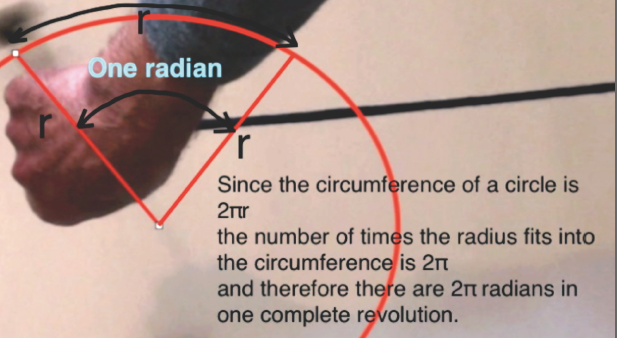The examples here are drawn from the video lesson below.

When a mass tied to a cord 0.16 metres long, is spun around and timed to make 2 revolutions each second. There are several ways of quoting how fast it is going. One way is just that; 2 revs per second

Another way is to give the speed in metres per second.  A mass moving in a circle is constantly changing direction. Since velocity is a vector (having direction as well as magnitude) it is constantly changing. However the size of the velocity (the speed) can be constant. We measure that in metres per second. Since the circumference is 1m the speed is 2ms

Angular velocity

One of the most common measurements in circular motion is the Angular Velocity. That is the angle that the mass rotates through each second. Since it makes two complete revolutions each one being 360 degrees that is 720 degrees per second. But in circular motion the most common angle measurement is not degrees but radians.

A radian, as you can see in the diagram, is the angle formed by three lines. Two of the lines are straight and one is the length of a radius but measured around the curve of the circle. If all the lines were straight then each internal angle would be 60 degrees but the angle formed here is slightly smaller. A radian is the angle at the centre of an arc. Each side is one radius and the length of the curve is also one radius. A radian is about 57.3 degrees.The circumference of a circle is 2Πr, in other words the radius fits into the circle 2Π times so there are 2Π radians in one complete turn.

In our example the mass rotates twice per second so that is 4Π radians per second which is equal to 12.56 radians per second.

The video lesson below may be useful in fitting these ideas together

Other pages related to circular motion are:

Force and acceleration in circular motion

Polar and geostationary satellites notes and video